Courses

# Solutions - Introduction and Types of solutions Class 12 Notes | EduRev

## Chemistry Class 12

Created by: Mohit Rajpoot

## JEE : Solutions - Introduction and Types of solutions Class 12 Notes | EduRev

The document Solutions - Introduction and Types of solutions Class 12 Notes | EduRev is a part of the JEE Course Chemistry Class 12.
All you need of JEE at this link: JEE

INTRODUCTION

The homogeneous mixture of two or more components is called a Solution.

Example

• A mixture of salt and sugar.
• A mixture of oxygen and nitrogen.
• A mixture of sugar in water.

HOMOGENEOUS AND HETEROGENEOUS MIXTURE
(i) Homogeneous mixture: A mixture of two or more components having no distinguish phase is called homogeneous mixture.
The homogeneous mixture is generally referred to as a solution.
Example: When two spoons of salt are mixed in a glass of water, the mixture so obtained has no distinguish phase and this is called a solution.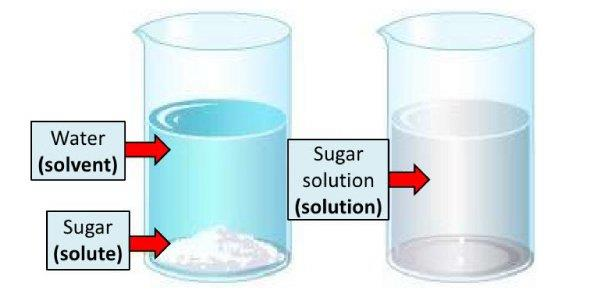Fig: Solution

Air is a mixture of different gases with no distinguish phase, thus, the air is often referred to as the solution.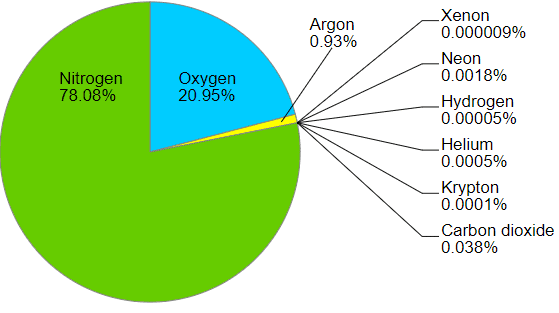In a homogeneous mixture, i.e. solution, the composition, and properties of the solution are uniform throughout.

(ii) Heterogeneous mixture: A mixture of two or more components with distinguish phase is called heterogeneous mixture.
Example: A mixture of mustard oil and water, in this mixture phases of water and mustard oil can be seen and distinguished clearly, and hence called heterogeneous mixture.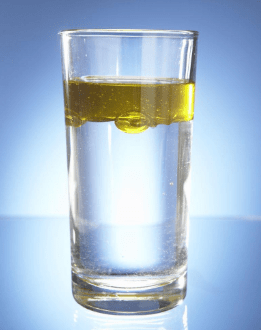Fig: Heterogeneous mixture: oil and water

SOLUTE AND SOLVENT

• Solvent: The substance which is present in the largest quantity in a solution; is generally referred to as a solvent.
Example: In the solution of salt in water, since water is present in larger quantity, thus, water is called solvent. It is the solvent that decides the phase of a solution.
• Solute: The substance which is present in lesser quantity in a solution is generally called solute.
In other words, one or more components present in a solution other than the solvent are is called solute.
Example: In the solution of salt in water, salt is called solute. The solute may be more than one.

Note: Physical state of solvent and solution is same.

BINARY SOLUTION
Solutions which contain only two components are called binary solution. For example, a solution of salt in water is a type of binary solution, as it contains only two components.

Binary Solution = Solute + Solvent

Similarly, it is called ternary and quaternary if it is composed of three and four components, respectively.
Thus; a solution may be regarded as a single phase containing more than one component.

DILUTE SOLUTION
A solution in which relatively a small amount of solute is dissolved in large amount of solvent is called a dilute solution.

CONCENTRATED SOLUTION
A solution in which relatively a large amount of the solute is present is called a concentrated solution.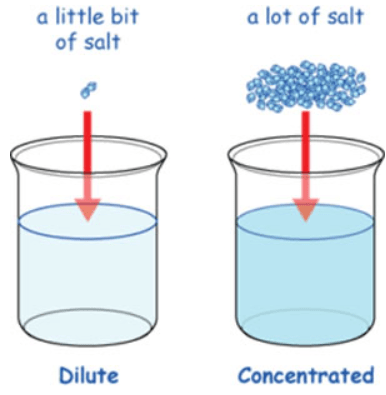Fig: Dilute and Concentrated Solution

PROPERTIES OF A SOLUTION

• A solution consists of a single phase i.e. it is a monophasic system.
• A solution is uniform throughout, such as uniform properties such as density, refractive index, etc.
• The components of a solution cannot be easily separated by physical methods.
• The composition of a solution is not definite but can vary within certain limits.
• Certain properties of solution such as density, viscosity, surface tension, boiling point, freezing point etc. vary with the composition of the solution.

TYPES OF SOLUTION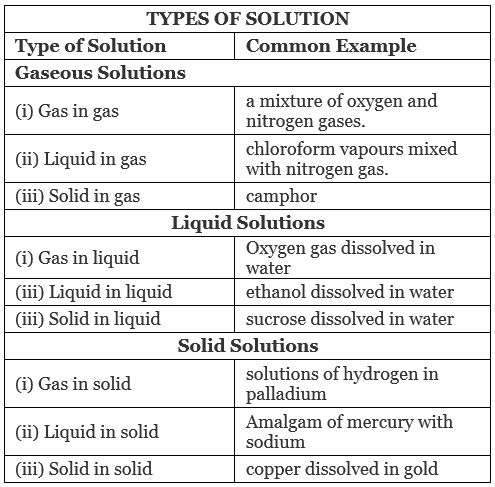In a solution, components may be solid, liquid or gas.

On this basis Solutions can be divided into the following three types:

1. Gaseous Solution: Solutions in which solvent is present is a gaseous state is called Gaseous solution.

Gaseous solutions can be divided into following three types on the basis of phases of solute and solvent;

(i) Gas - Gas Solution: Solutions in which solute and solvent both are gases; are called Gas-gas Solutions.
Example: solution (mixture) of nitrogen and oxygen, solution (mixture) of carbon dioxide and nitrogen, solution (mixture) of carbon dioxide and oxygen, etc.

(ii) Liquid Gas Solution: Solutions, in which solute is in liquid state and solvent is in a gaseous state, are called Liquid-Gas Solution.
Example: solution (mixture) of chloroform in nitrogen gas.

(iii) Solid Gas Solution: Solutions, in which solute is in solid state and solvent is in gasesous state, are called Solid-Gas Solutions.
Example: Solution (mixture) of camphor in nitrogen gas.

2. Liquid Solution: Solutions, in which solvent is present in liquid state, are called Liquid solutions.

Liquid solutions can be classified into following three types:
(i) Gas - Liquid Solution: Solutions having solute in gaseous state and solvent in liquid state, are called Gas - Liquid Solutions.
Example: Solution (mixture) of oxygen in water, mixture of carbon dioxide in water. Coca cola, a beverage, is an example of gas - liquid solution, as it has carbon dioxide dissolved in water.
(ii) Liquid - Liquid Solution: Solutions, in which solute and solvent both are present in liquid state, are called liquid liquid solutions.
Example: Vinegar solution, vinegar is the mixture of ethanoic acid and water. Solution of ethanol in water, etc.
(iii) Solid - Liquid Solution: Solutions, in which solutes present in solid state and solvent is in liquid state, are called solid liquid solutions.
Example: Solution of salt in water, solution of glucose in water, etc.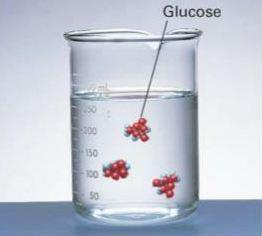Fig: Glucose in water

3. Solid Solution: Solutions having solvent in the solid state, are called solid solutions.

The solid solution can be divided into the following three categories:(i) Gas - Solid Solution: Solutions having solvent in solid state and solute in the gasesous state are called gas-solid solutions.
Example: solution of hydrogen in palladium.
(ii) Liquid - Solid Solution: Solutions having solvent in solid state and solute in the liquid state are called liquid-solid solutions.
Example: amalgam of mercury with sodium.
(iii) Solid - solid solution: Solutions having solvent and solute both in the solid state are called solid – solid solutions.
Example: Solution of gold and copper.

Offer running on EduRev: Apply code STAYHOME200 to get INR 200 off on our premium plan EduRev Infinity!

## Chemistry Class 12

255 videos|306 docs|201 tests

,

,

,

,

,

,

,

,

,

,

,

,

,

,

,

,

,

,

,

,

,

;